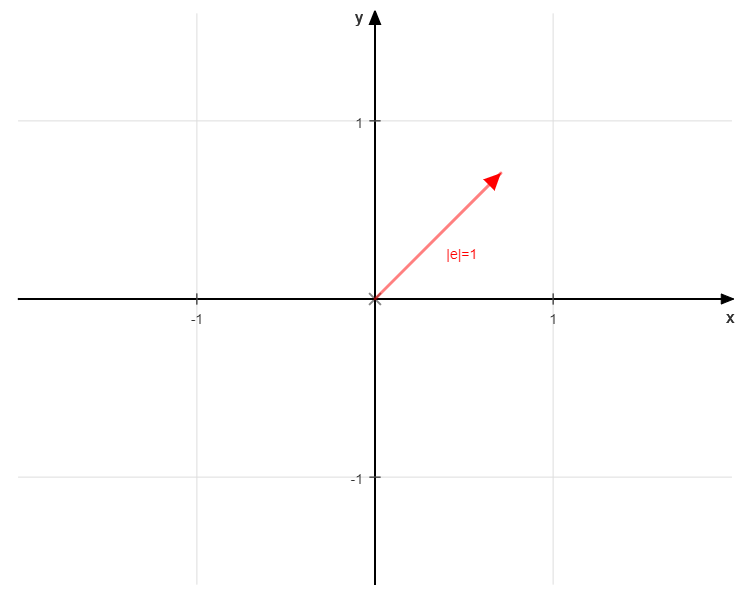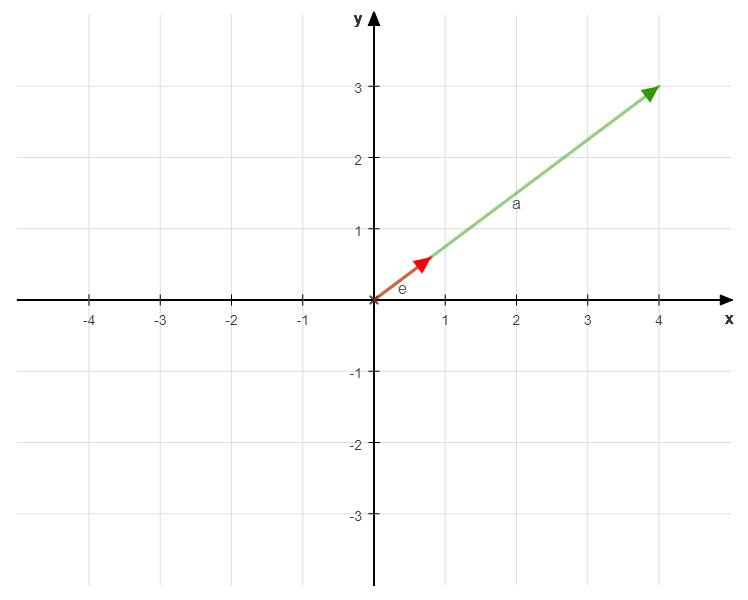# What is the application of unit vectors

## Unit vector

A vector with the uniform length is called a "unit vector". We mostly write for unit vector. The unit vector can thus be defined as:

We can calculate an associated unit vector for every vector (except for the zero vector with the length).

The unit vector formed points into the same direction like the vector. To identify the affiliation, the vector name is placed in the index of the unit vector:

The original vector is, its unit vector.If you multiply the unit vector by the amount of the original vector, you get this vector again.

### Calculation of the unit vector

The aim is to "normalize" the given vector, that is, to bring it to length.

To do this, we divide the vector (let's call it) by its length. Generally noted:

Calculation example:

The vector is given. The unit vector is now to be determined for this.

The formula for Calculation of the unit vector reads:

First we need to determine the vector length. This is calculated using the Pythagorean theorem:

Now we can insert the values ​​into our formula:

Let's draw the vector and its unit vector:We see that they are both pointing in the same direction.

So let's remember the:

General formula for calculating the unit vector (with components x and y):

### Length of the unit vector

Let's calculate the length of the unit vector from the above example to check whether its length is really:

Correctly. The length of the unit vector is.